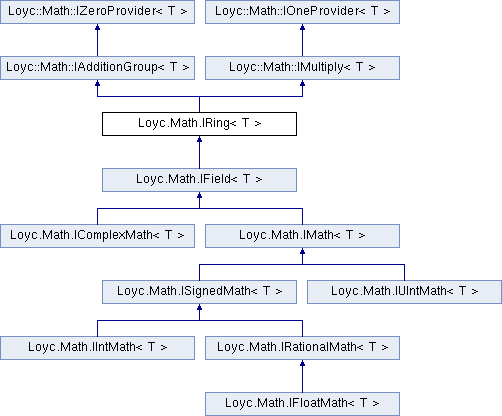Enhanced C# Language of your choice: library documentation
Loyc.Math.IRing< T > Interface Template Reference

This defines a Ring with the operations +,*
More...

Source file:
Inheritance diagram for Loyc.Math.IRing< T >:## Remarks

This defines a Ring with the operations +,*

Axioms that have to be satisified by the operations: The group axioms for + Associativity of *: a * (b*c) = (a*b) * c Neutral element of *: Multiply(One,a)==a for all a in T Distributivity: a * (b+c) = (a*b) + (a*c) (a+b) * c = (a*c) + (b*c)Properties inherited from Loyc.Math.IZeroProvider< T >
Zero` [get]`
Returns the "zero" or additive identity of this type. More...Properties inherited from Loyc.Math.IOneProvider< T >
One` [get]`
Returns the "one" or identity value of this type. More...Public Member Functions inherited from Loyc.Math.IAdditionGroup< T >Public Member Functions inherited from Loyc.Math.IMultiply< T >